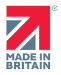CLOSE

# Understanding Power Factor Correction

Depending on the nature of electrical loads, considerable savings in electricity bills can be achieved through correcting power factor.

The active power of a load is drawn (and paid for) from the supply and is measured in kilowatts (kW). The apparent power of the load is the multiple of the current and the voltage (kVA). The ratio of the active power (kW) to the apparent power (kVA) is known as the power factor and referred to as cos ?.

In addition to the kilowatts per hour consumed, a charge is also made by the supply authority for the maximum kVAR demand on the supply. If the maximum kVAR can be reduced considerable savings can be made.

Reducing Power Factor

A reduction in power factor is achieved through the connection of suitable-sized capacitors to the supply to compensate for the reactive component of the load, reducing cos - closer to unity (which is 1.0). The nearer cos - is to unity, the less reactive power is drawn and therefore the greater the savings on utility bills.

In practice it is often uneconomic to improve power factor greater than 0.9 as the size of the capacitors would be extremely large and therefore the costs involved would outweigh any potential energy savings.

Power factor correction can be achieved through a dedicated system or fitted to one of Watford Control's EM range of voltage stabilisers (https://watfordcontrol.com/voltage-stabilisers/). This option can be realised through a fixed capacitor bank, which is suitable for applications where the load and power factor are fixed and are not likely to change. Alternately, the voltage stabiliser can be fitted with a power factor control system (https://watfordcontrol.com/power-factor-correction/) to automatically vary the correction capacitance in pre-set steps to ensure optimum power factor correction irrespective of load variations.

As previously mentioned, improving power factor will result in a reduction in the overall cost of electricity bills. For example, a three phase 100kW 400V load with a power factor of cos ?1 = 0.6 will draw a current of 242 amps per phase which is equal to a 167 kVA demand on the supply. Improving the power factor to cos ?2 = 1.0 the line current will be reduced to 144 amps reducing the load to 100kVA and in turn energy savings.

Watford Control have been solving power quality issues for over 70 years, including power factor. As such, we have designed a pfc calculator (https://watfordcontrol.com/power-factor-calculator/ ) which will allow you to understand the indicative cost of installing power factor correction or for more help please don?t hesitate to contact our experienced technical team (https://watfordcontrol.com/contact-us/).